Next: 5.3.1 Power Cosine Distribution Up: 5. Surface Rate Calculation Previous: 5.2.10 Benchmarks

# 5.3 Generation of Random Vectors

The generation of random directions is essential for the Monte Carlo based calculation of particle transport. It is difficult to find instructions for the generation of random directions which obey a certain angular distribution, except for uniform spherical  or cosine distributions . Therefore, recipes for the generation of variate distributions used in this work are given in the following discussion.

The arrival directions of particles at the source plane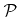and the directions of reemitted particles are usually described by probability densities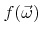, which only depend on the polar angle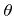relative to a certain direction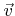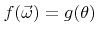with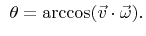(5.33)

Using spherical coordinates with respect tothe probability density function can be formulated as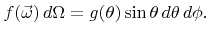(5.34)

Since this expression is separable, the azimuthal angle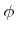and the polar angleare independent, which allows for the description of both variables by individual probability densities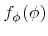and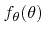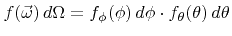with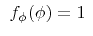and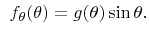(5.35)

The probability density function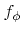is constant, which is a direct consequence of the rotational symmetry of the directional distribution. Therefore, since the azimuthal angle is uniformly distributed on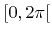, a random choice ofis trivial. Picking a random polar angle is more sophisticated. In the following sections algorithms are presented for selecting a polar angle according to directional distributions which are frequently used for the description of arrival or reemission angles.

SubsectionsNext: 5.3.1 Power Cosine Distribution Up: 5. Surface Rate Calculation Previous: 5.2.10 Benchmarks

Otmar Ertl: Numerical Methods for Topography Simulation